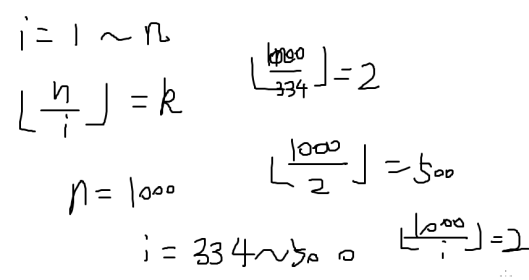## qbzt day2 下午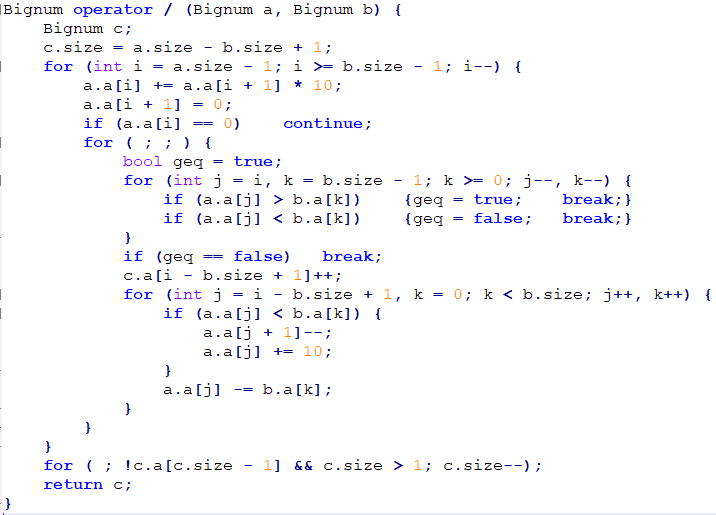87654.321-->08|76|54|.32|1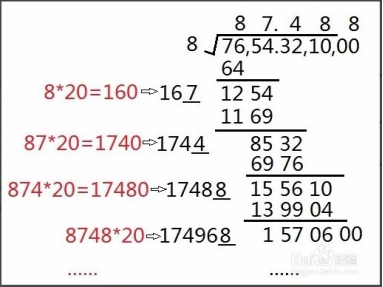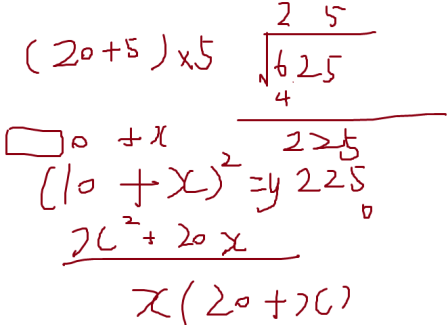（1）先把被开方的数由右到左每二位一组。（6,25)

（2）由左到右取每一组。（取的是6）

（3）取某数的平方，要比第一组数小，但某数+1的平方，要比第一组数大，这就是第一个开方值。（某数是2）

（4）把第一组数减去第一个开方值的平方，再取第二组数，构成余数。（6-2*2=2，余数为225）

（5）把第一个开方值*20，再加上估计的第二个开方值，它与第二个开方值相乘要比余数小，但把第一个开方值*20，再加上估计的第二个开方值+1，它与第二个开方值+1相乘要比余数大。（第二个开方值取5，2*20+5=45，45*5=225)

(a+b)^2=a^2+2ab+b^2=a^2+(2a+b)*b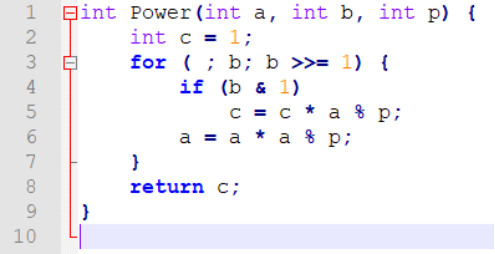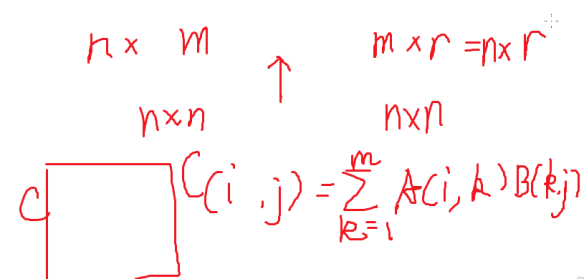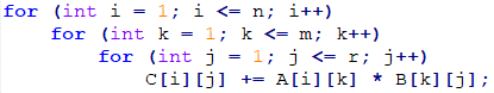1  1

1  0

[f[n],f[n-1]]*[矩阵]^k=[f[n+k],[n+k-1]]

[F(0) F(1)]T* Mn = [F(n) F(n+1)]T

F(n) = 7F(n-1) + 6F(n-2) + 5n + 4 * 3^n

[f[n-1,] f[n-2,] n, 3^n, 1]

[f[n], f[n-1], n+1, 3^(n+1) ,1]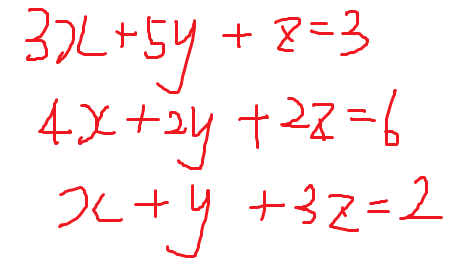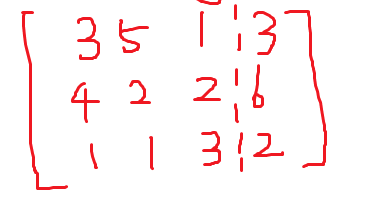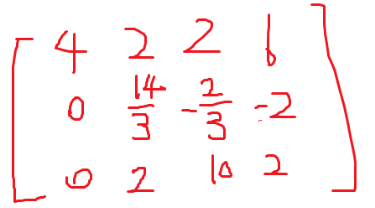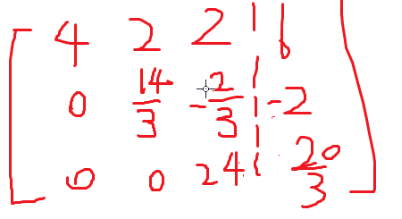筛法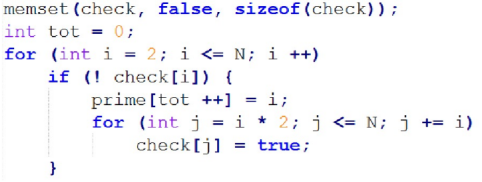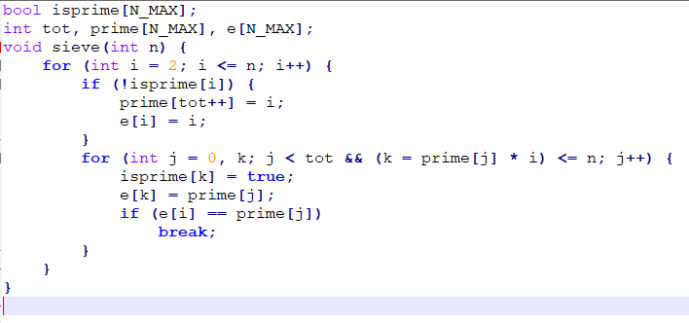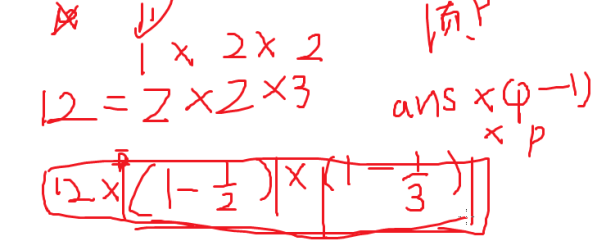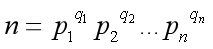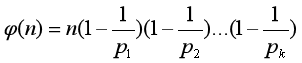1.i和p互素

Phi[i*p]=phi[i]*phi[p]

2.i的最小素因子刚好是p

Phi[i*p]=phi[i]*p

φ[i]=(p1-1)*p1^(q1-1)+(p2-1)*p2^(q2-1)......(pn-1)*pn^(qn-1)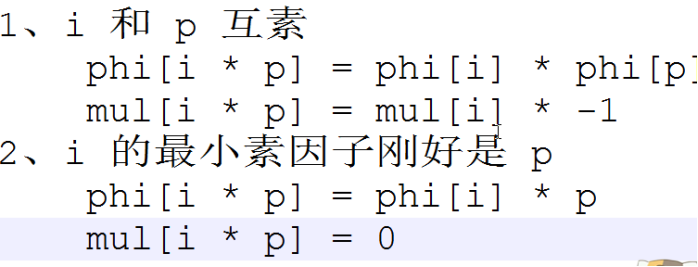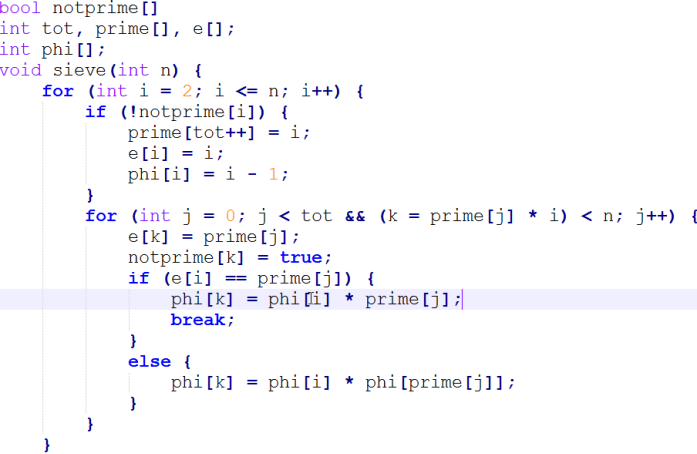1. i和p互素  mul[i*p]=mul[i]*(-1)
2. i的最小素因子刚好是p  mul[i*p]=0

1. k<sqrt(n)的时候，k只有sqrt(n)种取值

所以n div k的取值最多只有sqrt(n)种

div是整除

2.k>sqrt(n)的时候

n div k 显然小于sqrt(n)

所以它的取值也只有sqrt(n)种